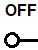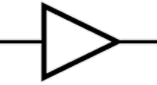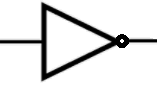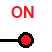﻿ Logic Gate Operation Demonstration Simulator ﻿# Logic Gate Operation Demonstration SimulatorThe logic gates include a buffer, an inverter, an AND gate, a NAND gate, an OR gate, a NOR gate, an XOR gate, and an XNOR gate.

### Buffer

The first logic gate is a buffer.

A buffer is a gate that outputs the same value that is input‌ into it.

Thus, if the input is 1, the output is 1. If the input is 0, the output is 0.The truth table for a buffer gate is shown below.
 Buffer Input Output 0 0 1 1

### Inverter

An inverter is a logic gate that will invert the input fed into it.

Thus, if a 1 is input, 0 is output. If a 0 is input, a 1 is output.The truth table for an inverter is shown below.

 Inverter Input Output 0 1 1 0

### AND Gate

An AND gate is a gate that will output a 1 only if all the inputs are 1.The truth table for the AND gate is shown below.

 AND gate Input Input Output 0 0 0 0 1 0 1 0 0 1 1 1

### NAND gate

The NAND gate represents an AND gate with an inverter attached to its output.

A NAND gate outputs a 1 only if all the inputs fed into it are 0.The truth table for a NAND gate is shown below.

 NAND gate Input Input Output 0 0 1 0 1 0 1 0 0 1 1 0

### OR gate

An OR gate is a gate that outputs a 1 if any of the inputs fed into it are 1.The OR gate is shown below.

 OR gate Input Input Output 0 0 0 0 1 1 1 0 1 1 1 1

### NOR gate

The NOR gate is an OR gate with an inverter attached to its output.The truth table for the NOR gate is shown below.
 NOR gate Input Input Output 0 0 1 0 1 0 1 0 0 1 1 0

### XOR gate

The XOR gate is a gate that outputs a 1 only if 1 or some of the inputs are 1 but not all.The truth table for the XOR gate is shown below.
 XOR gate Input Input Output 0 0 0 0 1 1 1 0 1 1 1 0

### XNOR gate

An XNOR gate is a NOR gate with an inverter attached to its output.The truth table for an XNOR gate is shown below.

 XNOR gate Input Input Output 0 0 1 0 1 0 1 0 0 1 1 1